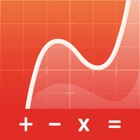Graphing Calculator Pro²

• SEARCH TYPE

All iPhone applications categories

All iPhone games categories# Graphing Calculator Pro²

7 Users
rating

## Screenshots

Description

FIVE REASONS WHY YOU NEED THIS APP
1. Keyboard and command syntax similar to physical graphing calculators -> No need to learn a new calculator.
2. Powerful emulator like Wabbitemu Calculator -> No more waiting to graph functions compared to slow physical handhelds.
3. Enter fractions, equations or functions in the same way as you would write them on paper.
5. High-resolution graphs

FULL GRAPHING CALCULATOR
• Graph more than ten functions at once, each with a different color
• Add Lines, Horizontals, Verticals, and Tangents to the graph
• Use your finger or the arrow keys to trace the coordinates of your functions
• Calculate the Zero, Minimum, Maximum, Intersection, and Derivative
• Find integrals and color the area under the function
• Set the X and Y coordinates of the graphing window; zoom in and out with your fingers

• Enter calculations in natural textbook format
• Includes all basic arithmetic (+, -, x, ÷, ^, ², √, π, …)
• Use advanced functions like sin, cos, tan, e, ln, Σ, ...
• Scroll your calculation history and modify existing calculations with one tab
• Store and recall variables

MATRIX CALCULATOR
• Work with matrices the easy way -> store them or enter them directly on the calculator interface
• Do basic matrix arithmetic operations like addition, subtraction, multiplication or power of a Matrix
• Put the matrix into Reduced Row-Echelon Form and solve linear equation systems
• Calculate the determinant, inversion or transpose the matrix

STATISTICS AND LISTS
• Use lists for all basic and trigonometric ​operations
• Enter lists using the Statistics List Editor or use curly brackets on the main screen
• NOTE: Some commands of Stat menu are currently under development

TUTORIAL AND CATALOG
• Easy to understand tutorial to get started
• Full Catalog of all commands with syntax, example, and possible error messages
• Tab on highlighted math terms to read on point definitions

PERFECT FOR HIGH SCHOOL & COLLEGE STUDENTS
• Recommended by teachers to use in math classes
• Pre-Algebra, Algebra 1 & 2
• Pre-Calculus, Calculus
• Permitted on SAT/ ACT/ AP (your phone might not be permitted, but you can use the app for homework and borrow an actual calculator from your school for the test)

## Users review

from 1.023 reviews

"Great"

7